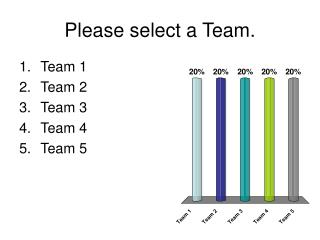DownloadDownload PresentationDownload Presentation- - - - - - - - - - - - - - - - - - - - - - - - - - - E N D - - - - - - - - - - - - - - - - - - - - - - - - - - -
##### Presentation Transcript

1. Please select a Team. • Team 1 • Team 2 • Team 3 • Team 4 • Team 5

2. Solve using square roots.

3. Solve using square roots.

4. A sports recreation company plans to manufacture a beach ball with a surface area of 7238 in.2 Find the radius of the beach ball. Use the formula , where A is the surface area and r is the radius of the sphere. • 24 in. • 48 in. • 75 in. • 576 in.

5. Team Scores

6. Solve the equation by factoring. Picture • z = 3 or z = 9 • z = 3 or z = –9 • z = –3 or z = 9 • z = –3 or z = –9

7. Solve by factoring • z = 1 or z = -2 • z = 1 or z = 2 • z = 3 or z = 9 • z = -3 or z = -9

8. Solve by factoring • c = 0 or c = -4 • c = 0 or c = 2 • c = 0 or c = 4 • c = 1 or c = -2

9. Team Scores

10. Factor to solve

11. Factor to solve.

12. The area of a playground is 336 yd2. The width of the playground is 5 yd longer than its length. Find the length and width of the playground. • length = 26 yd, width = 21 yd • length = 21 yd, width = 26 yd • length = 21 yd, width = 16 yd • length = 16 yd, width = 21 yd

13. Team Scores

14. Picture Find the number of real number solutions for the equation. • 2 • 0 • 1

15. Solve the equation using the method of your choice. Picture • 14, 4 • –4, –14 • 14, –14 • –4, 4

16. Team Scores

17. Find the missing value to complete the square. • 2 • 1 • 4 • 8

18. Complete the Square

19. Complete the Square

20. Team Scores

21. Complete the square

22. Use the Quadratic formula to solve

23. Hey, use the quadratic formula again!

24. Team Scores